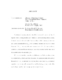## Abstract Elementary Classes with Lowenheim-Skolem Number Cofinal with Omega##### Files
umi-umd-5661.pdf(311.43 KB)
An abstract elementary class is a class $\aec$ of structures for some vocabulary $L$ together with a strong substructure'' relation $\prec_{\aec}$ on $\aec$ satisfying certain axioms. Abstract elementary classes include elementary classes with elementary substructure and classes axiomatizable in $L_{\infty,\omega}$ with elementary substructure relative to some fragment of $L_{\infty,\omega}$. For every abstract elementary class there is some number $\kappa$, called the L\"owenheim-Skolem number, so that every structure in the class has a strong substructure of cardinality $\leq \kappa$. We study abstract elementary classes with L\"owenheim-Skolem number $\kappa$, where $\kappa$ is cofinal with $\omega$, which have finite character. We generalize results obtained by Kueker for $\kappa=\omega$. In particular we show that $\aec$ is closed under $L_{\infty,\kappa}$-elementary equivalence and obtain sufficient conditions for $\aec$ to be $L_{\infty,\kappa}$-axiomatizable. The results depend on developing an appropriate concept of $\kappa$-a.e.